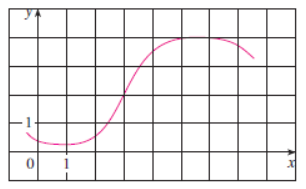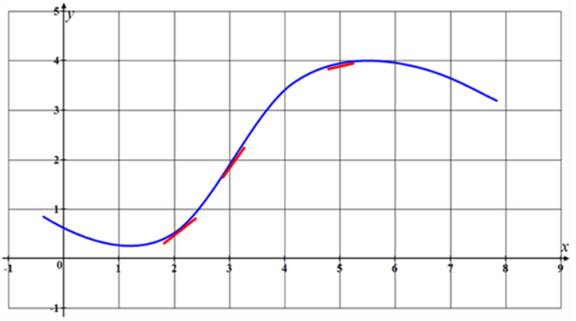# Thearrangement in increasing order for the given numbers.### Single Variable Calculus: Concepts...

4th Edition
James Stewart
Publisher: Cengage Learning
ISBN: 9781337687805### Single Variable Calculus: Concepts...

4th Edition
James Stewart
Publisher: Cengage Learning
ISBN: 9781337687805

#### Solutions

Chapter 2, Problem 29RE
To determine

## To find: Thearrangement in increasing order for the given numbers.

Expert Solution

The number can be arranged in increasing order as f''5<0<f'(5)<f'(2)<1<f'(3) .

### Explanation of Solution

Given information:

The given numbers are 01f'(2)f'(3)f'(5)f''(5) .

The given graph is shown in figure (1).Figure (1)

Calculation:

Using the given graph it observe that, the graph of f is a concave downword at the point 5 .

This implies that f''(x)<0 on the interval (3,9) .

So, f''(5)<0 .

The derivative of a function is the slope of the tangent line at that point.

So, draw the tangent line at the point 2,3 and 5 .Figure (2)

From the figure (2) observe that the graph f is increases at the point 2,3 and 5 .

So, f'(x)>0 at the point 2,3 and 5 .

That is, f'(2)>0,f'(3)>0, and f'(5)>0

The slope of the tangent line increases when the slope becomes sleeper.

The slope of the tangent line at x=3 is larger than the slope at x=2,5 , because the slope is sleeper at x=3 .

The slope of the tangent line at x=2 is larger than the slope at x=5 , because the slope is sleeper at x=2 .

Finally, The slope of the tangent line at x=5 is is closely appeared at horizontal line, the tangent line at x=5 has a small positive slope (nearly zero).

From the above discussion the number can be arranged as.

0<f'(5)<f'(2)<1<f'(3)

At f''5<0 .

The number are arranged as.

f''5<0<f'(5)<f'(2)<1<f'(3)

Therefore, the number can be arrangedin increasing order as f''5<0<f'(5)<f'(2)<1<f'(3) .

### Have a homework question?

Subscribe to bartleby learn! Ask subject matter experts 30 homework questions each month. Plus, you’ll have access to millions of step-by-step textbook answers!